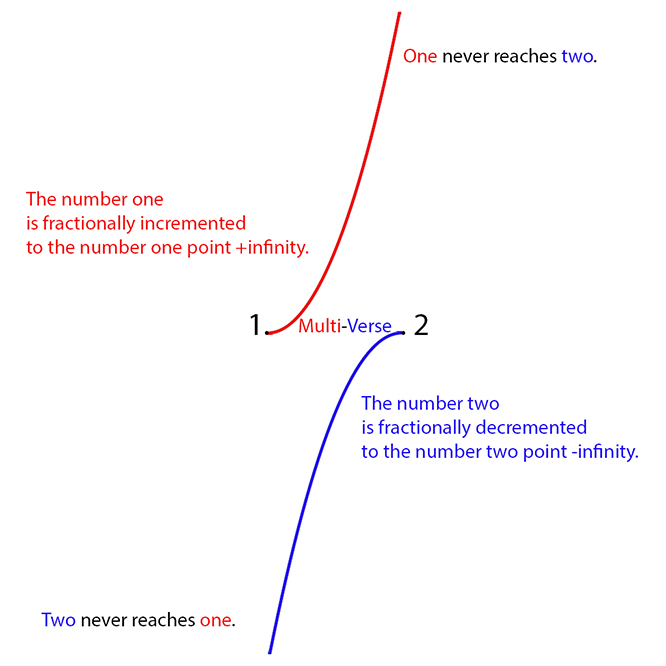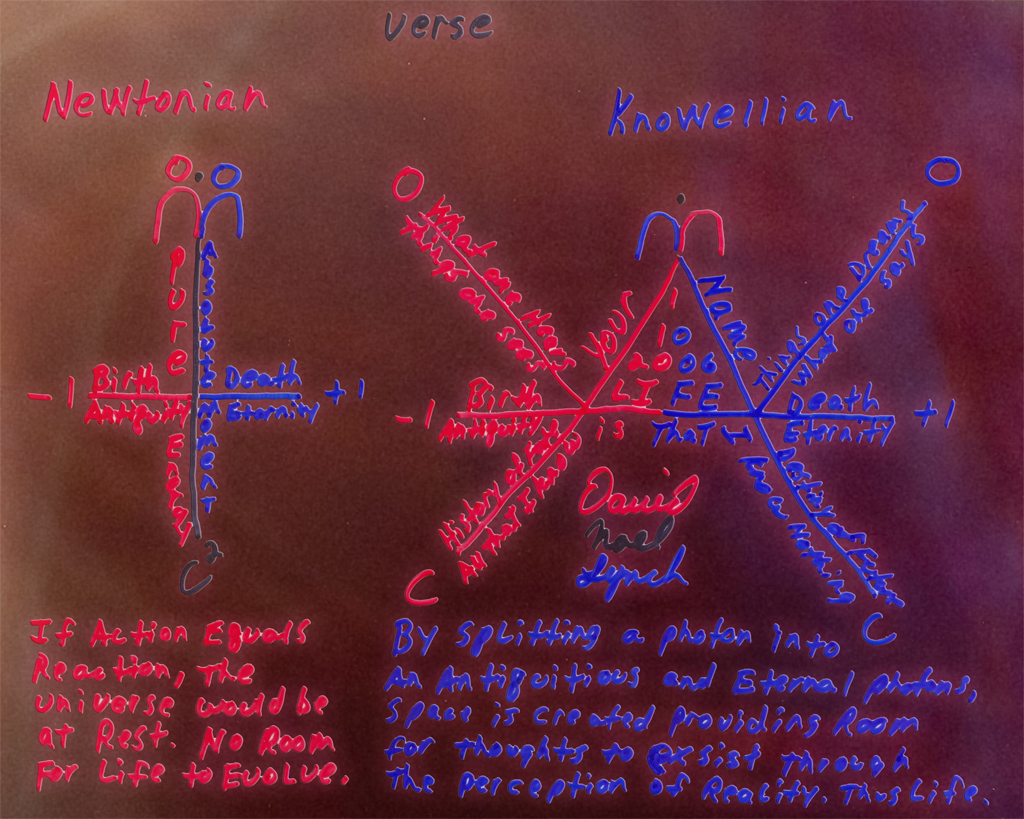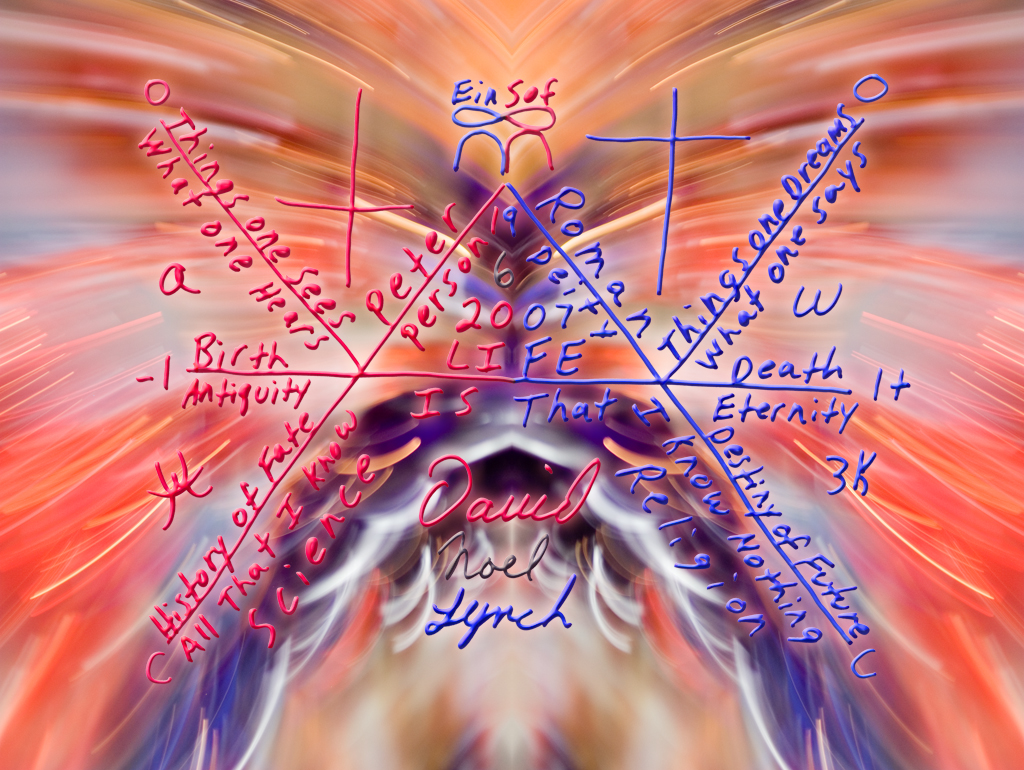From: David Lynch <dnl1960 at yahoo.com>
To:
Kok-Wei Bong <k.bong at griffith.edu.au>
Sent: Thursday, July 8, 2021, 11:23:43 PM EDT
Subject: god probably does play dice with the universe.

Kok-Wei,

Over the years I have reached out to those that might listen.

I could send you a barage of links to emails that I have sent to various scientific minds.
However; with all the ransomeware attacks, I doubt anyone would open my links.

The article,
"A strong no-go theorem on the Wigner’s friend paradox",
there are some interesting points.

If I may, what if the language spoken by Wigner and his associates is the problem?

That language being mathematics.

To resolve the anomalies created by the language of mathematics,
a new axiom is needed to eliminate many of the issues with Schrodinger's cat.

This new axiom of mathematics, “ -c>∞<c+ ”,
provides a solution to the problems created by the current axiom, -∞<0.0<∞+,
thus Science's greatest mistake is 0.0

Since web links are now land mines,
I have attached a couple images that show how the language of mathematics has lead brilliant minds astray.

The below image shows how the
multi~verse/many~worlds theories are a mathematical anomaly.http://lynchphoto.com/maudlin

Below is an graphic that shows how Newton's equation is inadequate to express the universe.http://lynchphoto.com/disney

Below is an equation that uses the logic of Lynch,
the energy of Einstein, the force of Newton,
and the sayings of Socrates to describe a moment of time as infinite.
I call the equation the KnoWell.http://lynchphoto.com/avignon

So until science adopts my new axiom of mathematics,
science will forever find rabbit holes.

I KnoWell, that my equation explains that the universe is in a steady state,
and that the observations attributed to the big bang are actually
the remnants of a chaos brane exchanging place with a control brane.

Thus: "The Emergence of the Universe is the Precipitation of Chaos through the Evaporation of Control."~3K

Regards,
David Noel Lynch